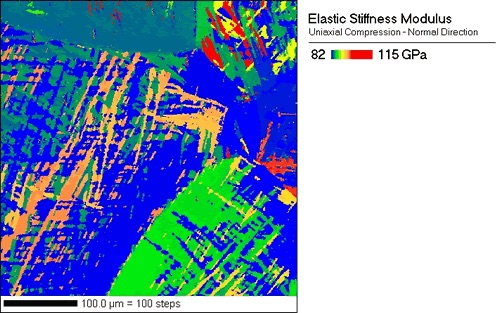# Ti Gal 4V cored from the face of a cast golf club driver

Collected By Matt Nowell, EDAXIn most polycrystals, the average elastic properties are assumed to be isotropic. However, it is well known that the elastic properties for a single crystal can be quite anisotropic.

The accompanying figure shows the variation in elastic stiffness due to differences in crystal orientation. The points in red are stiffer than points in blue. The material is titanium (Ti-Gal-4V) cored from the face of a cast golf club driver.

Elasticity theory allows stress and strain to be related through a stiffness tensor. Published values for single crystal elastic constants can be found in the literature. However, the single crystal elastic constants are given assuming the principle axes of the crystal are in coincidence with the principle axes of a cube. Using tensor algebra, the single crystal elastic constants can be determined for a crystal rotated from this ideal orientation. Furthermore, the elastic stiffness tensor can be reduced to a scalar elastic modulus by simply dividing the stress in a given sample direction by the strain in a particular sample direction. In the accompanying figure the elastic modulus has been calculated for the stress and strain normal to the sample surface. It is assumed that the applied force is in a direction normal to the sample surface.